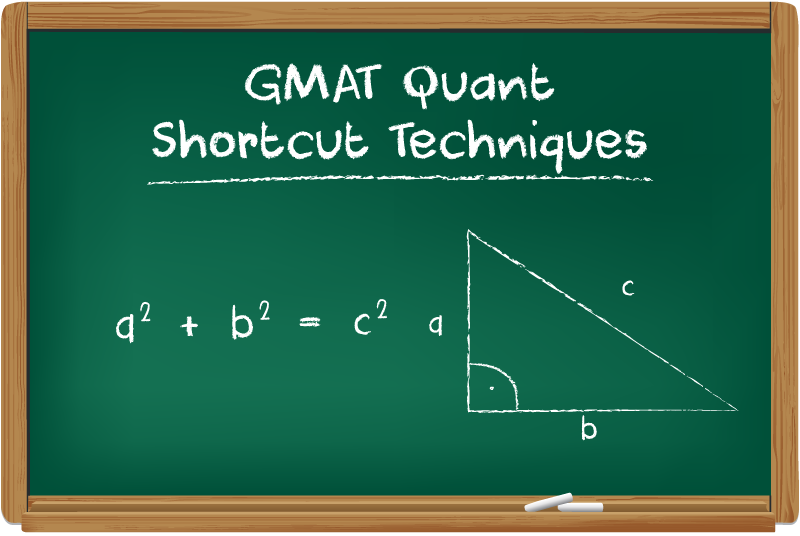# GMAT Quant Shortcut TechniquesThere’s no introduction required on why and how math shortcuts can save your time and energy in GMAT exam. In case you are not from maths background, or if you are a working professional and you lacks skills and time respectively to prepare for the exam, this post will definitely help you.

‘Patience’ and ‘Practice’ are the two Guru Mantras when it come to GMAT Preparation. Interesting fact about Maths is that if you practice everyday, within a month or two, you will be a master in GMAT Quant. So, pull up your socks and follow these simple Math techniques.

Tips to Speed up Mental Calculations

1) Memorize multiplication tables up to 35.

2) Learn square, square roots, cube & cube roots of numbers up to 50. Also, learn shortcut tricks to find square and cube of any number.

3) Avoid Calculators. Do all your day-to-day calculations mentally.

Note: Only knowing or understanding the following tricks does not assure your GMAT qualification. Along with these tricks, you need lots of GMAT mock test practice with application of these methods.

To add or subtract numbers which aren’t multiples of ten or hundred, find it’s nearest tens number and then add or subtract.

For example –

1) $172 + 47$

In case of $47$, the nearest ‘number with multiples of $10$’ is $50$

Hence, the calculation can be done as

$172 + (50-3)$

= $219$

2) $2285 – 192$

= $2285 – (200 – 8)$

= $2285 – 200 + 8$

= $2093$

2. Multiply or Divide by 5

To divide a number by $5$, double the number (i.e. multiply the number with $2$) and divide the result by $10$. The simple logic behind this trick is that it is easier to double a number and multiply or divide a number by $10$.

For example –

$\large \frac{93}{5}$

= $\large \frac{93 \times 2}{10}$

(Again at this stage, don’t waste time by multiplying 93 with 2, rather add $90 \times 2$ and $3 \times 2$, i.e. $180 + 6 = 186$)

= $\large \frac{186}{10}$

= $18.6$

Hence, the desired result of $93 \times 5$ is $18.6$

To multiply a number with $5$, just do the reverse process.

$88 \times 5$

= $\large \frac{88 \times 10}{2}$

= $\large\frac{880}{2}$

= $440$

3. Multiply Numbers Between 11 & 19

Example – $15 \times 13$

As all the numbers between 11 to 19 are multiples of 10, it is easier to break the above expression into four easy computations –

$15 + 3$;  $18 \times 10$;  $5 \times 3$;  $180 + 15$

Thus, $15 \times 13$ = $(18 \times 10) + (5 + 3)$= $195$

4. Square of Any Number Between 11 & 99

a) To find the square of $11$

Step 1 = $10$ is the nearest power of $10$ which can be taken as our base. Find out the deviation from this base.

$11 – 10 = 1$

Now, add this deviation with the number, i.e.

$11 + 1 = 12$

Step 2 = $1^{2}$ =  $1$

Hence, $11^{2} = 121$

b) To find the square of $14$

Step 1 = Find the deviation = $14 – 10$ = $4$

Add the deviation = $14 + 4$ = $18$

Step 2 = $4^{2}$ =  $16$

In this case when the square of the deviation is a two-digit number, you need to add the first digit with $18$.

i.e. $18 + 1$ = $19$

Hence, $14^{2} = 196$

5. Square of Any Number Above 100

a) To find the square of $106$

Step 1 = With base $100$, Find the deviation = $106 – 100$ = $6$

Add the deviation = $106 + 6$ = $112$

Step 2 = $6^{2}$ =  $36$

Hence, $106^{2} = 11236$

b) To find the square of $113$

Step 1 = Deviation is $113 – 100$ = $13$

Now, add this deviation with the number, i.e.

$113 + 13$ = $126$

Step 2 = Find Square of the deviation = $13^{2}$ =  $169$

In this case when the square of the deviation is a three-digit number, you need to add the first digit with $126$.

i.e. $126 + 1$ = $127$

Hence, $113^{2} = 12769$

We’ll be glad to help you in your GMAT preparation journey. You can ask for any assistance related to GMAT and MBA from us by calling us at +918884544444. You can write to us at gmat@byjus.com.

Read more GMAT Quant related articles.